## Thursday, July 18, 2013

### Blend modes

source: http://en.wikipedia.org/wiki/Blend_modes

# Blend modes

determine how two layers are blended into each other.## Normal blend mode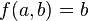$f(a, b) = b$

## Dissolve

The dissolve mode takes random pixels from both layers

## Multiply and Screen

### Multiply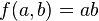$f(a,b) = ab$
For image editing it is sometimes more convenient to simply go to the Curves dialog of the software, as it gives more flexibility in the shape of the curves.

### Screen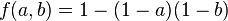$f(a,b) = 1 - (1 - a)(1 - b)$
This yields an inverse effect from multiply. The result is a brighter picture.

### Overlay

Overlay combines Multiply and Screen blend modes. The parts of the top layer where base layer is light become lighter, the parts where the base layer is dark become darker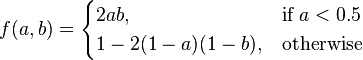$f(a,b) = \begin{cases} 2 a b, &\mbox{if } a < 0.5 \\ 1 - 2 ( 1 - a ) ( 1 - b ), &\mbox{otherwise} \end{cases}$

### Hard Light

Hard Light combines Multiply and Screen blend modes. Equivalent to Overlay, but with the bottom and top images swapped.

### Soft Light

This is a softer version of Hard Light.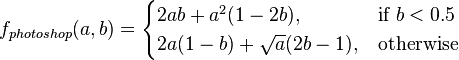$f_{photoshop}(a,b) = \begin{cases} 2 a b + a^2 (1 - 2 b), &\mbox{if } b < 0.5 \\ 2 a (1 - b) + \sqrt{a} (2 b - 1), &\mbox{otherwise} \end{cases}$

## Dodge and burn

Dodge and burn change the lightness of the pictures, inspired by the dodging and burning performed in a darkroom.

• Dodge modes:
• The Screen blend mode inverts both layers, multiplies them, and then inverts that result.  f(a, b) = 1 - (1-a)(1-b)
• The Color Dodge blend mode divides the bottom layer by the inverted top layer. This lightens the bottom layer depending on the value of the top layer: the brighter the top layer, the more its color affects the bottom layer. Blending with white gives white. Blending with black does not change the image.  f(a, b) = b / (1-a)
• The Linear Dodge (Add) blend mode simply sums the values in the two layers. Blending with white gives white. Blending with black does not change the image. f(a, b) = a+b
• Burn modes:
• The Multiply mode simply multiplies each component in the two layers.  f(a, b) = a*b
• The Color Burn mode divides the inverted bottom layer by the top layer, and then inverts the result. This darkens the top layer increasing the contrast to reflect the color of the bottom layer. The darker the bottom layer, the more its color is used. Blending with white produces no difference.  f(a, b) = 1- (1-b)/a
• The Linear Burn mode sums the value in the two layers and subtracts 1. This is the same as inverting each layer, adding them together (as in Linear Dodge), and then inverting the result. Blending with white leaves the image unchanged. f(a, b) = (a+b) - 1
• Vivid Light: this blend mode combines Color Dodge and Color Burn (rescaled so that neutral colors become middle gray). Dodge applies when values in the top layer are lighter than middle gray, and burn to darker values. The middle gray is the neutral color. When color is lighter than this, this effectively moves the white point of the bottom layer down by twice the difference; when it is darker, the black point is moved up by twice the difference. (The perceived contrast increases.)
• Linear Light: this blend mode combines Linear Dodge and Linear Burn (rescaled so that neutral colors become middle gray). Dodge applies to values of top layer lighter than middle gray, and burn to darker values. The calculation simplifies to the sum of bottom layer and twice the top layer, subtract 1. The contrast decreases.

## Simple arithmetic blend modes

### Divide

This blend mode simply divides pixel values of one layer with the other, but it's useful for brightening photos if the color is on grey or less.

This blend mode simply adds pixel values of one layer with the other. In case of values above 1 (in the case of RGB), white is displayed.

### Subtract

This blend mode simply subtracts pixel values of one layer with the other. In case of negative values, black is displayed.

### Difference

Difference subtracts the top layer from the bottom layer or the other way round, to always get a positive value. Blending with black produces no change, as values for all colors are 0. (The RGB value for black is 0,0,0). Blending with white inverts the picture.
One of the main utilities for this is during the editing process, when it can be used to verify alignment of pictures with similar content. Exclusion is a very similar blend mode with lower contrast.

### Darken Only

Darken Only creates a resultant pixel that retains the smallest components of the foreground and background pixels. If the foreground pixel has the components r1g1, and b1, and the background has r2g2b2, the resultant pixel is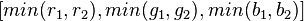$[min(r_1, r_2), min(g_1, g_2), min(b_1, b_2)]$

### Lighten Only

Lighten Only has the opposite action of Darken Only. It selects the maximum of each component from the foreground and background pixels. The mathematical expression for Lighten Only is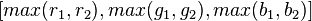$[max(r_1, r_2), max(g_1, g_2), max(b_1, b_2)]$

## Hue, saturation and luminosity

Photoshop’s huesaturationcolor, and luminosity blend modes are based on a color space with dimensions that the article HSL and HSV calls hue, chroma, and luma.

Because these blend modes are based on a color space which is much closer than RGB to perceptually relevant dimensions, it can be used to correct the color of an image without altering perceived lightness, and to manipulate lightness contrast without changing the hue or chroma. The Luminosity mode is commonly used for image sharpening, because human vision is much more sensitive to fine-scale lightness contrast than color contrast.